Processing ......FreeComputerBooks.com Links to Free Computer, Mathematics, Technical Books all over the World

Logics of Time and Computation
GIS Visualizer - Geographic Data Visualized on 40+ Maps! Click here for details.
• Title Logics of Time and Computation
• Author(s) Robert Goldblatt
• Publisher: Center for the Study of Language and Inf; 1 edition (September 1, 1992)
• Hardcover/Paperback: 180 pages
• eBook PDF (200 pages, 6.8 MB)
• Language: English
• ISBN-10: 0937073946
• ISBN-13: 978-0937073940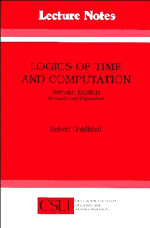Book Description

This is a short but excellent introduction to modal, temporal, and dynamic logic, etc. It manages to cover, in highly readable style, the basic completeness, decidability, and expressability results in a variety of logics of the three kinds considered.

Sets out the basic theory of normal modal and temporal propositional logics; applies this theory to logics of discrete (integer), dense (rational), and continuous (real) time, to the temporal logic of henceforth, next, and until, and to the propositional dynamic logic of regular programs.

• Robert Goldblatt is a mathematical logician who is Emeritus Professor in the School of Mathematics and Statistics at Victoria University, Wellington, New Zealand.
Reviews, Ratings, and Recommendations: Related Book Categories: Read and Download Links:Similar Books:
•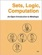Sets, Logic, Computation: An Open Introduction to Metalogic

It covers naive set theory, first-order logic, sequent calculus and natural deduction, the completeness, compactness, and Löwenheim-Skolem theorems, Turing machines, and the undecidability of the halting problem and of first-order logic.

•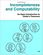Incompleteness and Computability: Gödel's Theorems

This book is an introduction to metamathematics and Gödel's Theorems. It covers recursive function theory, arithmetization of syntax, the first and second incompleteness theorem, models of arithmetic, second-order logic, and the lambda calculus.

•Introduction to Mathematical Logic (Vilnis Detlovs, et al)

This book explores the principal topics of mathematical logic. It covers propositional logic, first-order logic, first-order number theory, axiomatic set theory, and the theory of computability. Discusses the major results of Gödel, Church, Kleene, Rosser, and Turing.

•A Friendly Introduction to Mathematical Logic (Chris Leary)

In this user-friendly book, readers with no previous study in the field are introduced to the basics of model theory, proof theory, and computability theory, leading to rigorous proofs of Gödel's First and Second Incompleteness Theorems.

•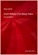Gödel Without (Too Many) Tears (Peter Smith)

How is this remarkable result of Gödel's Theorems established? This short book explains. The aim is to make the Theorems available, clearly and accessibly, even to those with a quite limited formal background.

•What is Mathematics: Godel's Theorem and Around (K. Podnieks)

This accessible book gives a new, detailed and elementary explanation of the Godel incompleteness theorems and presents the Chaitin results and their relation to the da Costa-Doria results, which are given in full, but with no technicalities.

•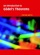An Introduction to Gödel’s Theorems (Peter Smith)

The formal explanations are interwoven with discussions of the wider significance of the two Gödel's Theorems. It will be of equal interest to mathematics students taking a first course in mathematical logic.

Book Categories
 :All CategoriesTop Free BooksRecent BooksMiscellaneous BooksComputer EngineeringComputer LanguagesComputer ScienceData Science/DatabasesJava and Java EE (J2EE)Linux and UnixMathematicsMicrosoft and .NETMobile ComputingNetworking and CommunicationsSoftware EngineeringSpecial TopicsWeb Programming
Other Categories